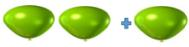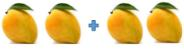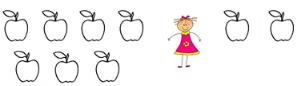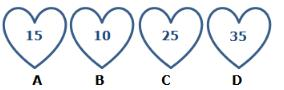Courses

# Test: Addition - 2

## 20 Questions MCQ Test Mathematics (Maths) for Class 1 | Test: Addition - 2

Description
This mock test of Test: Addition - 2 for Class 1 helps you for every Class 1 entrance exam. This contains 20 Multiple Choice Questions for Class 1 Test: Addition - 2 (mcq) to study with solutions a complete question bank. The solved questions answers in this Test: Addition - 2 quiz give you a good mix of easy questions and tough questions. Class 1 students definitely take this Test: Addition - 2 exercise for a better result in the exam. You can find other Test: Addition - 2 extra questions, long questions & short questions for Class 1 on EduRev as well by searching above.
QUESTION: 1

Solution:
QUESTION: 2

Solution:
QUESTION: 3

### Which of the following represent 40?

Solution:
QUESTION: 4

Add and match : 2 + 1, by counting number of stars in option

Solution:
QUESTION: 5

Add and match : 1 + 1

Solution:
QUESTION: 6

Which of the following equals fifteen?

Solution:
QUESTION: 7

Add : 5 + 4 = ________

Solution:
QUESTION: 8

Add : 9 + 2 = _______

Solution:
QUESTION: 9

Which of the following sign will make the sentence true?
16         2    =    14

Solution:

The '-' sign will make the equation true. 16 - 2 = 14.

QUESTION: 10

Count and add the picturesSolution:
QUESTION: 11

Count and add the picturesSolution:
QUESTION: 12

There are 7 apples in basket. Radha puts 2 more apples in it. How many apples are there in the basket?Solution:
QUESTION: 13

Write the missing numerals : 3 + _____ = 9

Solution:
QUESTION: 14

Write the missing numerals : 7 + ____ = 15

Solution:
QUESTION: 15

Which of the following options add up to 45?Solution:
QUESTION: 16

Aaron has 8 boxes and Adam has 6 boxes. How many boxes do they have together?

Solution:
QUESTION: 17

Jack has 6 bananas and Olivia has 2 bananas. How many bananas do they have together?

Solution:
QUESTION: 18

Which of the following have a sum of 18?

Solution:

9 + 9 = 18

QUESTION: 19

Add and match : 3 + 2

Solution:
QUESTION: 20

Add and match : 2 + 2

Solution: